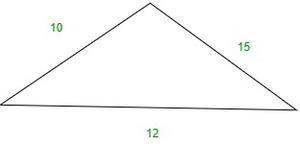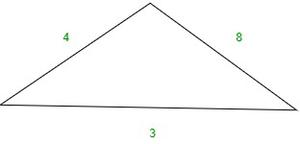GeeksforGeeks App
Open AppBrowser
Continue

# How to determine if an angle of a triangle is acute or obtuse?

Geometry has been a part of ancient history as well as the modern world. At present time geometry is being used for designing, constructional works, architecture in choice of material for construction, and so on. It is equally a part of the technical world as geometry is being used for computing various designs, manufacturing, creating blueprints, programming, etc.

Geometry is a study of mathematics that deals with the study of shapes and their properties. The term is was derived from Greek words ‘ge’ and ‘materia’ which means earth and measurement respectively.

The early approach to geometry can be observed in their construction and architectural works of ancient times from the use of various shapes in a very specific way.

### Angles

Angles can be defined as is the space between two intersecting lines meeting at a certain point. The components of an angle include two arms known as sides of the angle and a meeting point where angle forms called a vertex. Angles are measured in degrees which measures from 0 degrees to 360 degrees.

The first angle was supposed by Carpus of Antioch.

An Angle can be simply defined as a shape or space formed at the meeting point of two intersecting rays. The term angle was derived from the Latin word ‘angulus’ which means ‘a corner.

Angles are divided into different types on the basis of measurement.

### How to determine if an angle of a triangle is acute or obtuse?

If a triangle does not have a specific mention of a right angle. Then, we can determine if a triangle is acute, right, or obtuse by using the converse Pythagorean theorem.

As if the sum of the square of the two shortest sides of a triangle is greater than the square of the longest side. The triangle is an acute triangle.

a2+b2>c2

Let’s see this with a mathematical example.Here,

=>a2+b2=(10)2+(12)2

=>a2+b2=100+144

=>a2+b2=244

=>c2=(15)2

=>c2=225

Since 244>225, and by the relation a2+b2>c the given triangle is acute.

Similarly, if the sum of the square of the two shorter sides of a triangle is smaller than the square of the longest side. The triangle is an obtuse triangle.

a2+b2<c2Here,

=>a2+b2=(4)2+(3)2

=>a2+b2=16+9

=>a2+b2=25

=>c2=(8)2

=>c=64

Since, 64>25, and by the relation a2+b2<c2 the given triangle is obtuse.

### Sample Questions

Question 1. What is an obtuse angle triangle?

An obtuse triangle is a triangle that has one obtuse angle (greater than 90°) and two acute angles.

Question 2. How many acute angles are there in an acute triangle?